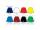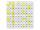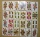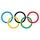Digits

How many odd four-digit numbers can we create from digits: 0, 3,5,6,7?

Result

n =  300

Solution:Leave us a comment of example and its solution (i.e. if it is still somewhat unclear...):Be the first to comment!To solve this example are needed these knowledge from mathematics:

Would you like to compute count of combinations?

Next similar examples:

1. Divisibility 2How many divisors has integer number 13?
2. Football teamFootball team has in store black, purple and orange shirts, blue and white shorts and striped and gray socks. How many different outfits players may start?
3. The resultHow many times I decrease the number 1632 to get the result 24?
4. Number unknownAdela thought the two-digit number, she added it to its ten times and got 407. What number does she think?
5. Write decimalsWrite in the decimal system the short and advanced form of these numbers: a) four thousand seventy-nine b) five hundred and one thousand six hundred and ten c) nine million twenty-sixAdded together and write as decimal number: LXVII + MLXIV
7. Roman numeralsWrite numbers written in Roman numerals as decimal.
8. SubtractionTest what do you know about the subtraction of two numbers: a) make a difference if the minuend 4,307,288 and subtrahend is 472008 b) minuend is 4000 more than subtrahend. What's the difference? c) the difference is 38900 less than the minuend. What is th
9. Seven timesWhich number seven times is just as higher as 27, how much is smaller than 29?
10. DivisionWhich number in division 16 give 12 and the rest 3?
11. Primes 2Which prime numbers is number 2025 divisible?
12. Roman numerals 2-Subtract up the number written in Roman numerals. Write the results as Roman numbers.
13. What is missing (1000)What number is to add to get 1000?
14. NumberDetermine unknown number if you know that difference between five times and triple of number is 42.
15. MultiplesWhat is the sum of the multiples of number 7 that are greater than 30 but less than 56?
16. CirclesFor the circle c1(S1; r1=146 cm) and c2(S2; r2 = 144 cm) is distance of centers |S1S2| = 295 cm. Determine the distance between the circles.
17. If-then equationIf 5x - 17 = -x + 7, then x =